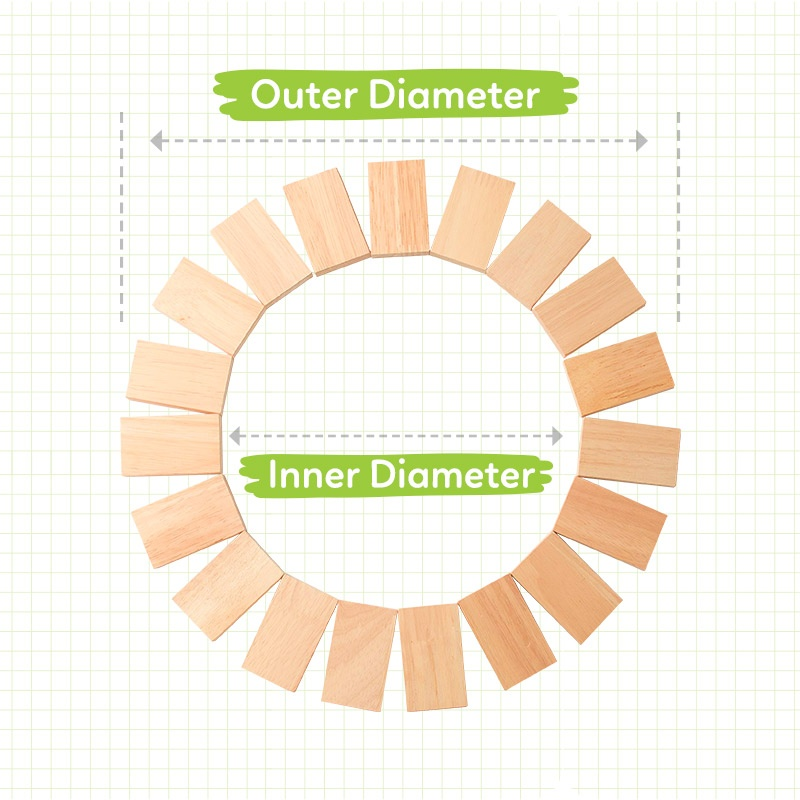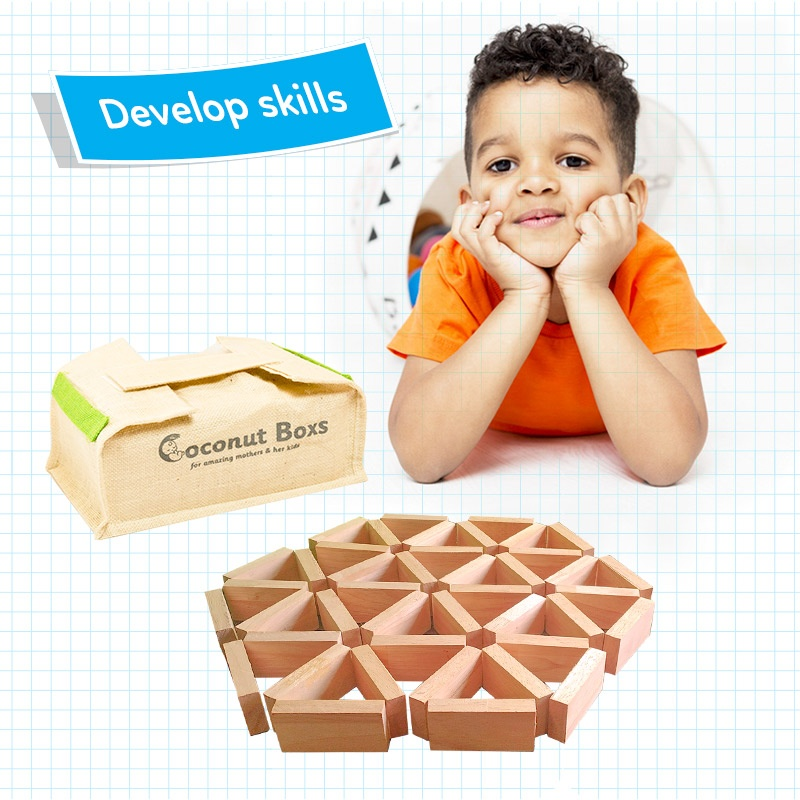### Boring Mathematics

Let’s take the example below.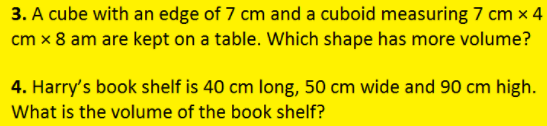These types of exercises in mathematics are boring because there is nothing interesting. There is nothing to discover and wonder about. No excitement. Children are to just follow the standard instructions and formula. This is the primary reason mathematics learning is one of the most boring activities in school.

Let’s give a life to the same problem and make children creative. Let them wonder about it. Let them discover something new. Here we make it flexible for children to use his own method, their own imagination and strategy.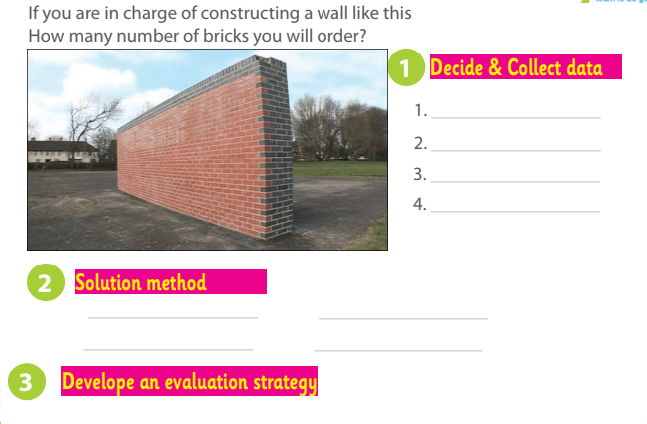Children have a chance to discover something on their own. Something to discuss and learn from each other. Something to touch and feel. Something connected and relevant to their daily life.

### Mathematics is meaningless

Let’s look at the usual way algebra is taught to our children.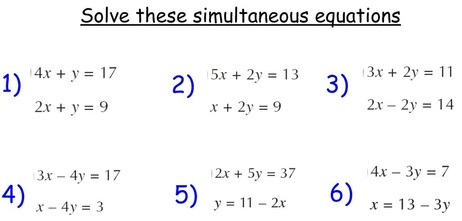Does it make sense to our kids? We removed the interesting parts and its representation to practical objects. We stripped all the mathematics from the problem and just presented mere notation. This stream of meaningless mathematics kills the children’s curiosity to learn. The beauty of mathematics is made into meaningless junk in the name of teaching the methods. There is nothing wrong in learning the techniques in solving mathematical problems. But if we remove the “why” part of mathematics and just focus on “what” to do, we are making mathematics meaningless for children.

The same algebraic problem can be introduced in a way children wonder and discover the mathematics behind it. Let’s look at the following example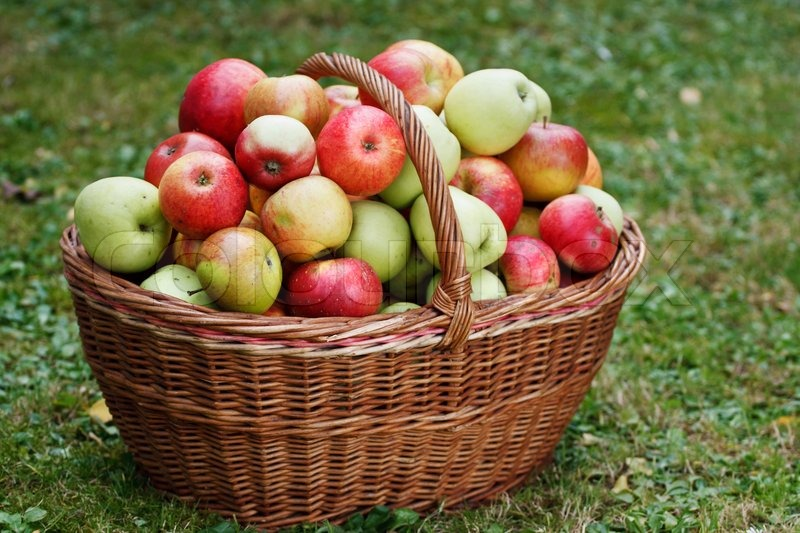How many apples are there in the basket? Find it without actually counting. Find an easy way!

Let’s say the number of apples in the basket as x. Since at present we do not know how many, we call it with a notation x. Let us keep the weight of an apple as y. Later we will find the exact value of y.

If we measure the total weight of all apples and say it turned out 80 kg.

If we multiply the number of apples by the weight of an apple we will get the total weight. Let us express it x multiplied by y equals total weight.

So x y = 80

Now how to find the values of x,y
Let us take 10 apples and find their weight as 4 kg

So 10 y =4 If we solve these sets of equations, we can find the values of x (number of apples in the basket).

Some curious children may ask, each apple has varying weight. How do you account for this? That is where the concept of an average comes. Now if we want to prove our prediction with a confidence interval, then introduce the concept of standard deviation and probability distribution. Meaningful mathematics is blended with every other concept in mathematics and leads to deeper understanding. On the other hand, stripped-down, meaningless exercises are cruel jokes on children.

### Mathematics = Fear

Most of us still remember the fear mathematics unleashed on. Especially the proofs in geometry. Geometry is a wonderful branch of mathematics, but the way it is taught made learners run away from mathematics.

Imagine the following problem. The jargon associated with it removes the original objective of appreciating mathematics in the problem. At the same time, the enforcement of obtaining a proof at an early stage of learning leaves little room for curiosity looking at the nature of mathematical ideas.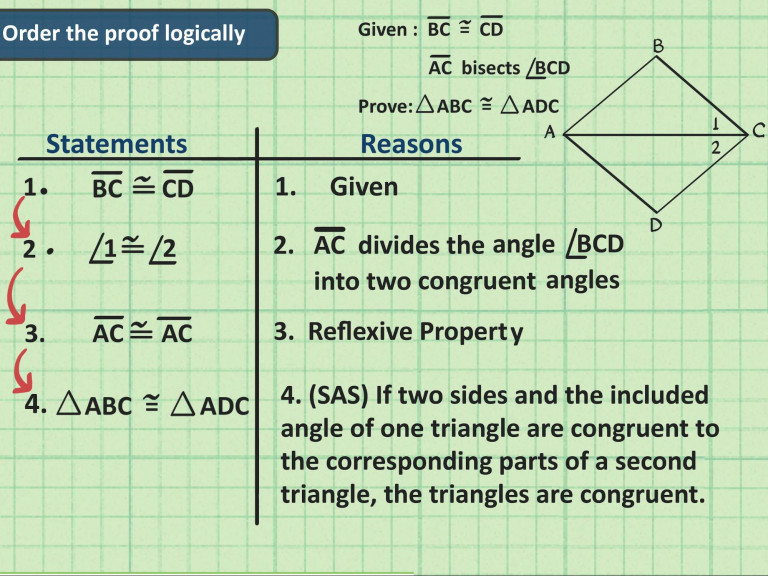Instead, let us give an opportunity to engage joyfully the geometrical ideas. By sheer imagination and experimenting with simple rectangular blocks, students can learn many insights of geometry.